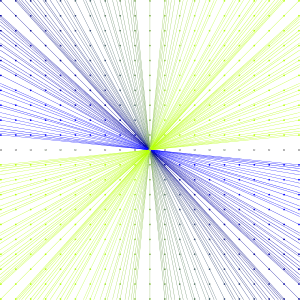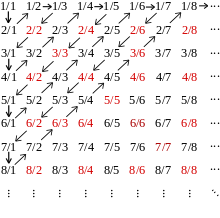# Rational number

"Rationals" redirects here. For other uses, see Rational (disambiguation).The rational numbers (ℚ) are included in the real numbers, and in turn include the integers (ℤ), which include the natural numbers (ℕ)

In mathematics, a rational number is any number that can be expressed as the quotient or fraction p/q of two integers, a numerator p and a non-zero denominator q. Since q may be equal to 1, every integer is a rational number. The set of all rational numbers, often referred to as "the rationals", is usually denoted by a boldface Q (or blackboard bold, Unicode ); it was thus denoted in 1895 by Giuseppe Peano after quoziente, Italian for "quotient".

The decimal expansion of a rational number always either terminates after a finite number of digits or begins to repeat the same finite sequence of digits over and over. Moreover, any repeating or terminating decimal represents a rational number. These statements hold true not just for base 10, but also for any other integer base (e.g. binary, hexadecimal).

A real number that is not rational is called irrational. Irrational numbers include 2, π, e, and φ. The decimal expansion of an irrational number continues without repeating. Since the set of rational numbers is countable, and the set of real numbers is uncountable, almost all real numbers are irrational.

The rational numbers can be formally defined as the equivalence classes of the quotient set (Z × (Z \ {0})) / ~, where the cartesian product Z × (Z \ {0}) is the set of all ordered pairs (m,n) where m and n are integers, n is not 0 (n ≠ 0), and "~" is the equivalence relation defined by (m1,n1) ~ (m2,n2) if, and only if, m1n2m2n1 = 0.

In abstract algebra, the rational numbers together with certain operations of addition and multiplication form the archetypical field of characteristic zero. As such, it is characterized as having no proper subfield or, alternatively, being the field of fractions for the ring of integers. Finite extensions of Q are called algebraic number fields, and the algebraic closure of Q is the field of algebraic numbers.

In mathematical analysis, the rational numbers form a dense subset of the real numbers. The real numbers can be constructed from the rational numbers by completion, using Cauchy sequences, Dedekind cuts, or infinite decimals.

Zero divided by any other integer equals zero; therefore, zero is a rational number (but division by zero is undefined).

## Terminology

The term rational in reference to the set Q refers to the fact that a rational number represents a ratio of two integers. In mathematics, "rational" is often used as a noun abbreviating "rational number". The adjective rational sometimes means that the coefficients are rational numbers. For example, a rational point is a point with rational coordinates (that is a point whose coordinates are rational numbers; a rational matrix is a matrix of rational numbers; a rational polynomial may be a polynomial with rational coefficients, although the term "polynomial over the rationals" is generally preferred, for avoiding confusion with "rational expression" and "rational function" (a polynomial is a rational expression and defines a rational function, even if its coefficients are not rational numbers). However, a rational curve is not a curve defined over the rationals, but a curve which can be parameterized by rational functions.

## Arithmetic

### Embedding of integers

Any integer n can be expressed as the rational number n/1.

### Equalityif and only if### Ordering

Where both denominators are positive:if and only ifIf either denominator is negative, the fractions must first be converted into equivalent forms with positive denominators, through the equations:andTwo fractions are added as follows:### Subtraction### Multiplication

The rule for multiplication is:### Division

Where c ≠ 0:Note that division is equivalent to multiplying by the reciprocal of the divisor fraction:### Inverse

Additive and multiplicative inverses exist in the rational numbers:### Exponentiation to integer power

If n is a non-negative integer, thenand (if a ≠ 0):## Continued fraction representation

Main article: Continued fraction

A finite continued fraction is an expression such aswhere an are integers. Every rational number a/b has two closely related expressions as a finite continued fraction, whose coefficients an can be determined by applying the Euclidean algorithm to (a,b).

## Other representations

• common fraction:• mixed numeral:• repeating decimal using a vinculum:• repeating decimal using parentheses:• continued fraction using traditional typography:• continued fraction in abbreviated notation: [2; 1, 2]
• egyptian fraction:• prime power decomposition:• quote notation: 3!6

are different ways to represent the same rational value.

## Formal constructionA diagram showing a representation of the equivalent classes of pairs of integers

Mathematically we may construct the rational numbers as equivalence classes of ordered pairs of integers (m,n), with n ≠ 0. This space of equivalence classes is the quotient space (Z × (Z \ {0})) / ~, where (m1,n1) ~ (m2,n2) if, and only if, m1n2m2n1 = 0. We can define addition and multiplication of these pairs with the following rules:and, if m2 ≠ 0, division byThe equivalence relation (m1,n1) ~ (m2,n2) if, and only if, m1n2m2n1 = 0 is a congruence relation, i.e. it is compatible with the addition and multiplication defined above, and we may define Q to be the quotient set (Z × (Z \ {0})) / ~, i.e. we identify two pairs (m1,n1) and (m2,n2) if they are equivalent in the above sense. (This construction can be carried out in any integral domain: see field of fractions.) We denote by [(m1,n1)] the equivalence class containing (m1,n1). If (m1,n1) ~ (m2,n2) then, by definition, (m1,n1) belongs to [(m2,n2)] and (m2,n2) belongs to [(m1,n1)]; in this case we can write [(m1,n1)] = [(m2,n2)]. Given any equivalence class [(m,n)] there are a countably infinite number of representation, sinceThe canonical choice for [(m,n)] is chosen so that n is positive and gcd(m,n) = 1, i.e. m and n share no common factors, i.e. m and n are coprime. For example, we would write [(1,2)] instead of [(2,4)] or [(−12,−24)], even though [(1,2)] = [(2,4)] = [(−12,−24)].

We can also define a total order on Q. Let ∧ be the and-symbol and ∨ be the or-symbol. We say that [(m1,n1)] [(m2,n2)] if:The integers may be considered to be rational numbers by the embedding that maps m to [(m,1)].

## PropertiesA diagram illustrating the countability of the rationals

The set Q, together with the addition and multiplication operations shown above, forms a field, the field of fractions of the integers Z.

The rationals are the smallest field with characteristic zero: every other field of characteristic zero contains a copy of Q. The rational numbers are therefore the prime field for characteristic zero.

The algebraic closure of Q, i.e. the field of roots of rational polynomials, is the algebraic numbers.

The set of all rational numbers is countable. Since the set of all real numbers is uncountable, we say that almost all real numbers are irrational, in the sense of Lebesgue measure, i.e. the set of rational numbers is a null set.

The rationals are a densely ordered set: between any two rationals, there sits another one, and, therefore, infinitely many other ones. For example, for any two fractions such that(whereare positive), we haveAny totally ordered set which is countable, dense (in the above sense), and has no least or greatest element is order isomorphic to the rational numbers.

## Real numbers and topological properties

The rationals are a dense subset of the real numbers: every real number has rational numbers arbitrarily close to it. A related property is that rational numbers are the only numbers with finite expansions as regular continued fractions.

By virtue of their order, the rationals carry an order topology. The rational numbers, as a subspace of the real numbers, also carry a subspace topology. The rational numbers form a metric space by using the absolute difference metric d(x,y) = |xy|, and this yields a third topology on Q. All three topologies coincide and turn the rationals into a topological field. The rational numbers are an important example of a space which is not locally compact. The rationals are characterized topologically as the unique countable metrizable space without isolated points. The space is also totally disconnected. The rational numbers do not form a complete metric space; the real numbers are the completion of Q under the metric d(x,y) = |xy|, above.

In addition to the absolute value metric mentioned above, there are other metrics which turn Q into a topological field:

Let p be a prime number and for any non-zero integer a, let |a|p = pn, where pn is the highest power of p dividing a.

In addition set |0|p = 0. For any rational number a/b, we set |a/b|p = |a|p / |b|p.

Then dp(x,y) = |xy|p defines a metric on Q.

The metric space (Q,dp) is not complete, and its completion is the p-adic number field Qp. Ostrowski's theorem states that any non-trivial absolute value on the rational numbers Q is equivalent to either the usual real absolute value or a p-adic absolute value.